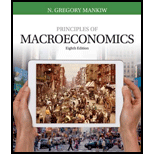# Suppose that this year’s money supply is $500 billion, nominal GDP is$10 trillion, and real GDP is $5 trillion. a. What is the price level? What is the velocity of money? b. Suppose that velocity is constant and the economy’s output of goods and services rises by 5 percent each year. What will happen to nominal GDP and the price level next year if the Fed keeps the money supply constant? c. What money supply should the Fed set next year if it wants to keep the price level stable? d. What money supply should the Fed set next year if it wants inflation of 10 percent?BuyFindarrow_forward ### Principles of Macroeconomics (Mind... 8th Edition N. Gregory Mankiw Publisher: Cengage Learning ISBN: 9781305971509 #### Solutions Chapter SectionBuyFindarrow_forward ### Principles of Macroeconomics (Mind... 8th Edition N. Gregory Mankiw Publisher: Cengage Learning ISBN: 9781305971509 Chapter 17, Problem 1PA Textbook Problem 2365 views ## Suppose that this year’s money supply is$500 billion, nominal GDP is $10 trillion, and real GDP is$5 trillion.a. What is the price level? What is the velocity of money?b. Suppose that velocity is constant and the economy’s output of goods and services rises by 5 percent each year. What will happen to nominal GDP and the price level next year if the Fed keeps the money supply constant?c. What money supply should the Fed set next year if it wants to keep the price level stable?d. What money supply should the Fed set next year if it wants inflation of 10 percent?

Subpart (a):

To determine
Money supply, price level, and velocity.

### Explanation of Solution

The velocity is calculated as follows:

Velocity = Nominal GDPMoney supply=$10,000 billion$500 billion=20

Velocity is 20

Subpart (b):

To determine
Money supply, price level, and velocity.

Subpart (c):

To determine
Money supply, price level, and velocity.

Subpart (d):

To determine
Money supply, price level, and velocity.

### Still sussing out bartleby?

Check out a sample textbook solution.

See a sample solution

#### The Solution to Your Study Problems

Bartleby provides explanations to thousands of textbook problems written by our experts, many with advanced degrees!

Get Started